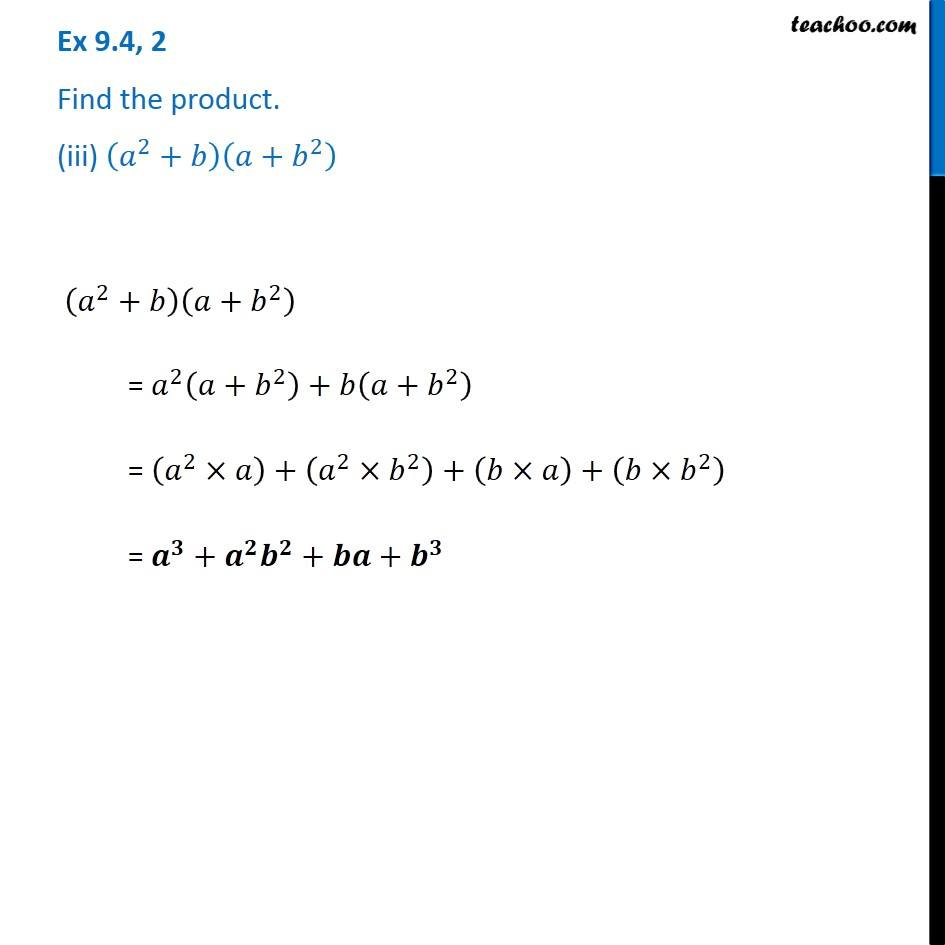Subscribe to our Youtube Channel - https://you.tube/teachoo

1. Chapter 9 Class 8 Algebraic Expressions and Identities
2. Concept wise
3. Multiplication of Polynoimals by Polynomials

Transcript

Ex 9.4, 2 Find the product. (iii) (𝑎^2+𝑏)(𝑎+𝑏^2 ) (𝑎^2+𝑏)(𝑎+𝑏^2 ) = 𝑎^2 (𝑎+𝑏^2 )+𝑏(𝑎+𝑏^2 ) = (𝑎^2×𝑎)+(𝑎^2×𝑏^2 )+(𝑏×𝑎)+(𝑏×𝑏^2 ) = 𝒂^𝟑+𝒂^𝟐 𝒃^𝟐+𝒃𝒂+𝒃^𝟑

Multiplication of Polynoimals by Polynomials

About the AuthorDavneet Singh
Davneet Singh is a graduate from Indian Institute of Technology, Kanpur. He has been teaching from the past 9 years. He provides courses for Maths and Science at Teachoo.